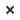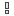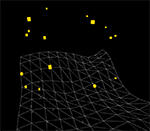# Collision detection between particles and polygonsClick to run demo

A basic example of collision detection in 3D, where moving particles bounce off a static terrain.

A couple things on it which could be of use ...

(A) To find out where a moving particle hits a polygon boils down to asking the question, "Where does a ray intersects a plane?" One or two Google searches will give you some math and some code examples... The gist is that the particle plus its velocity vector gives you the "ray" in question; the plane that it does or does not intersect is the one created by the triangle of the polygon you're testing against.

In this case, with the polygon which makes up the 'terrain', the triangles are set at regular intervals on the X/Z plane (in Papervision3D, an instance of "Plane3D"), so it was relatively straightforward to find the right triangle to do a "hit test" on. If it's a more irregularly shaped polygon, you'll have to iterate thru all the triangles testing for the occurrence of an intersection, or find clever ways of minimizing the number of tests you have to perform on the polygon).

(B) When a collision has been detected, you have to figure out the direction of the bounce. The solution I arrived at comes from asking the question, "How do you rotate a point or vector around an arbitrary axis?" (Again, Google is your friend). In this case, that arbitrary axis is the normal of the triangle that the particle has hit. The velocity vector of the particle is reversed (since, after all, the particle has to "bounce") and rotated 180 degrees. The result of this is that the angle between the new vector and the triangle normal equals the angle between the original vector and the triangle normal.